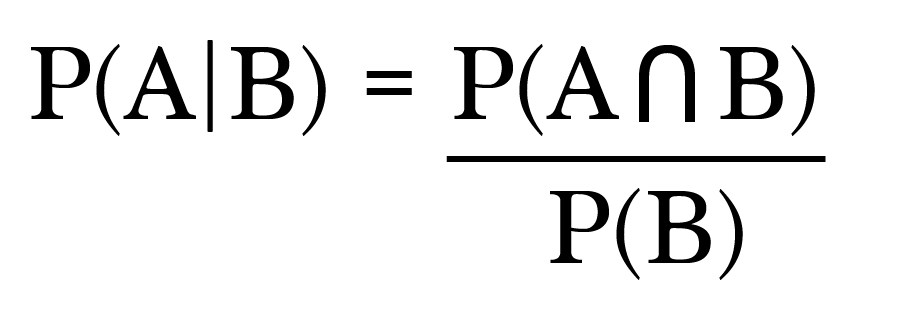The Monty Hall Game Show Problem

Bayes’ Theorem

Ah, the good ole Monty Hall Game Show problem. Such a classic. This is one of those problems that makes me feel all warm and fuzzy inside whenever I think about it. That probably has to do with the fact that it reminds me of this scene from one of my all time favorite movies, “21.”

Isn’t that just the best scene? I seriously love that movie. Anyhow, back to the math. Let’s break down exactly what Ben is talking about.

Some Assumptions

To fully understand this problem, we first have to understand exactly what’s going on here. We assume that the host of the game show knows exactly which door the car is behind.

After the player chooses a door, the host will always choose a different door that reveals a goat.

And lastly, we must assume that whether or not the player has correctly chosen the door with the car, the host always offers the opportunity for the player to switch to the remaining door.

What is Bayes’ Theorem?

You can think of Bayes’ Theorem as a handy little math trick. Basically, if you want to compute the outcome of a conditional probability, you can use the probability of other related events to figure it out.

Let’s derive Bayes’ Theorem to see how it works.

Deriving Bayes’ Theorem

Suppose we’d like to evaluate the probability of an event A happening given that some other event B has already happened. In math notation we write this as P(A|B) (read: “probability of A given B”).

A basic rule of conditional probability states that the P(A|B) is equivalent to the following:Read: Probability of A given B is equivalent to the probability of A and B divided by the probability of B.

Multiply both sides by P(B):

Do the same with P(B|A).

** Remember a basic rule in probability is that:

Therefore, we can set result 1 and result 2 equal to each other:

Lastly, divide both sides by P(B) and we now have an alternative method for calculating the P(A|B), which is Bayes’ Theorem.

The Monty Hall Solution

Alright, let’s start by defining a few events:

• Event A: The event of winning the car by choosing door A.
• Event B: The event of winning the car by choosing door B.
• Event C: The event of winning the car by choosing door C.

At this point, we know that all the events have an equal probability of 1/3.

Let’s say you choose door A and then the host opens door B to reveal a goat. Let’s pause here and calculate a few probabilities that will come in handy later.

Let’s call the event that the host opens door B, HB. Now, let’s calculate the different probabilities of HB given events A, B and C above.

How do we know this? Well, if the car is truly behind door A (event A), then the host may open door B or door C, yielding a 1/2 probability the host chooses door B.

Of course if the car is behind door B, then the host cannot open door B because the host must open a door with a goat behind it.

Lastly, if the car is behind door C and you already chose door A, the host must open door B, yielding a probability of 1 for this scenario.

To switch or not?

Okay, so now we’re ready to compute the probabilities of sticking with door A, which is the P(A|HB), and switching to door C, P(C|HB), using Bayes’ theorem of course.

P(A|HB)

Probability of winning a car if you stick to door A, given the host opens door B:

Applying Bayes’ Theorem we have:

Now the P(HB) is really the total of three different scenarios:

• Probability the host chooses B given the car is behind A = P(HB|A)•P(A)
• Probability the host chooses B given the car is behind B = P(HB|B)•P(B)
• Probability the host chooses B given the car is behind C = P(HB|C)•P(C)

Let’s rewrite our equation, using the sum of the above in place of P(HB):

This really looks worse than it actually is. The good news is we already know all the probabilities we need to do this calculation. So I’m going to replace each probability with the values we calculated earlier in this post and solve.

Alright, so we have a 1/3 chance of winning if we stay with door A.

P(C|HB)

Probability of winning a car if you switch to door C, given host opens door B:

Again, using Bayes’ Theorem we get:

Substitute in the appropriate values and simplify.

The Result

You have a significantly better chance of winning by switching to the other door because the probability of winning by staying is 1/3 versus winning by switching which is 2/3.

And that’s all there is to it :)